# Samacheer Kalvi 12th Business Maths Solutions Chapter 10 Operations Research Ex 10.2

Students can download 12th Business Maths Chapter 10 Operations Research Ex 10.2 Questions and Answers, Samacheer Kalvi 12th Business Maths Book Solutions Guide Pdf helps you to revise the complete Tamilnadu State Board New Syllabus and score more marks in your examinations.

## Tamilnadu Samacheer Kalvi 12th Business Maths Solutions Chapter 10 Operations Research Ex 10.2

Question 1.
What is the Assignment problem?
Solution:
Suppose that we have ‘m’ jobs to be performed on ‘n’ machines. The cost of assigning each job to each machine is Cij. (i = 1, 2,…, n and j = 1, 2,…. n).Our objective is to assign different jobs to different machines (one job per machine) to minimize the overall cost. This is known as the assignment problem.

Question 2.
Give the mathematical form of the assignment problem.
Solution:
The mathematical form of assignment problem is Minimize $$\mathrm{Z}=\sum_{i=1}^{n} \sum_{j=1}^{n} \mathrm{C}_{i j} x_{i j}$$
Subject to the constraints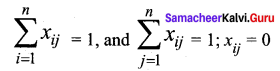(or) 1 for all i = 1, 2, …….. n and j = 1, 2, …….. n
where Cij is the cost of assigning ith job to jth machine and xij represents the assignment of ith job to jth machine.Question 3.
What is the difference between Assignment Problem and Transportation Problem?
Solution:
The assignment problem is a special case of the transportation problem. The differences are given below.

 Transportation Problem Assignment Problem 1. This is about reducing the cost of transportation merchandise 1. This is about assigning finite sources to finite destinations where only one destination is allotted for one source with a minimum cost 2. Number of sources and number of demand need not be equal 2. Number of sources and the number of destinations must be equal 3. If total demand and total supply are not equal then the problem is said to be unbalanced. 3. If the number of rows is not equal to the number of columns then problems are unbalanced. 4. It requires 2 stages to solve: Getting initial basic feasible solution, by NWC, LCM, VAM and optimal solution by MODI method 4. It has only one stage. Hungarian method is sufficient for obtaining an optimal solution

Question 4.
Three jobs A, B, and C one to be assigned to three machines U, V, and W. The processing cost for each job machine combination is shown in the matrix given below. Determine the allocation that minimizes the overall processing cost. (cost is in ₹ per unit)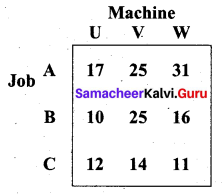Solution:
Here the number of rows and columns are equal.
the given assignment problem is balance.
Step 1: We select the smallest element from each row and subtract from other elements in its row.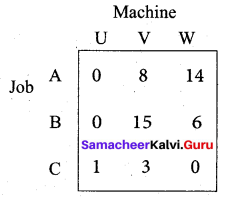Column V has no zero. Go to step 2.
Step 2: Select the smallest element from each column and subtract from other elements in its column.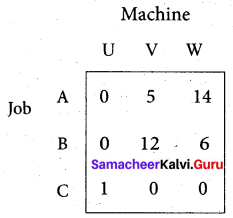Since each row and column contains at least one zero, assignments can be made.
Step 3: (Assignment)
Row A contains exactly one zero. We mark it by □ and other zeros in its column by x.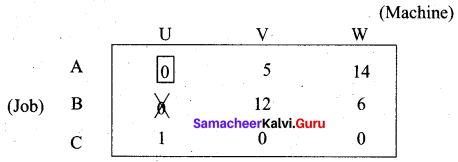Now proceed column-wise. Column V has exactly one zero. Mark by □ and other zeros in its row by X.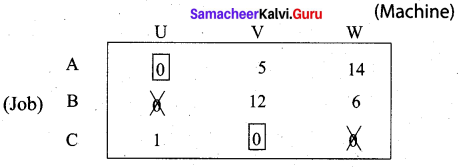Now there is no zero in row B to assign the job. So proceed as follows. Draw a minimum number of lines to cover all the zeros in the reduced matrix. Subtract 5 from all the uncovered elements and add to the element at the intersection of 2 lines as shown below.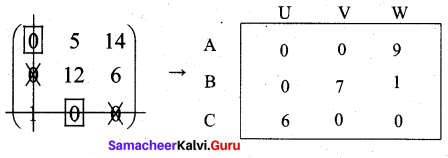Now start the whole procedure once again for the assignment to get the following matrix.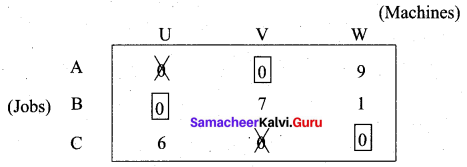Thus all the 3 assignments have been made. The optimal assignment schedule and the total cost is
Job Machine Cost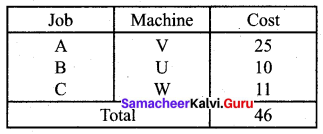Question 5.
A computer centre has got three expert programmers. The centre needs three application programmes to be developed. The head of the computer centre, after studying carefully the programmes to be developed, estimates the computer time in minutes required by the experts to the application programme as follows.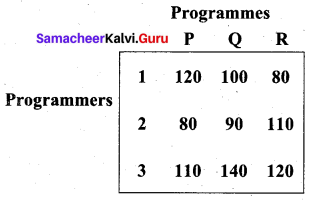Assign the programmers to the programme in such a way that the total computer time is the least.
Solution:
Here the number of rows equals the number of columns. So the given problem is balanced and we can find a solution.
Step 1: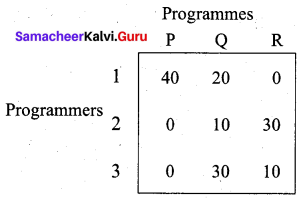Step 2: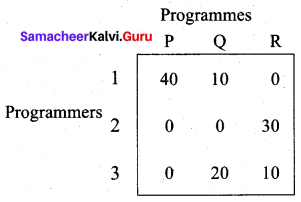Step 3: (Assignment)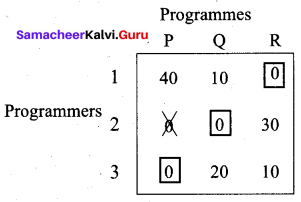Now all the 3 programmes have been assigned to the programmers. The optimal assignment schedule and the total cost is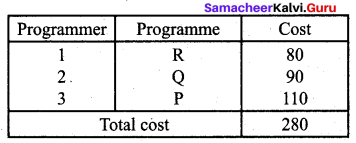The optimal assignment (minimum) cost is ₹ 280.

Question 6.
A departmental head has four subordinates and four tasks to be performed. The subordinates differ in inefficiency and the tasks differ in their intrinsic difficulty. His estimates of the time each man would take to perform each task is given below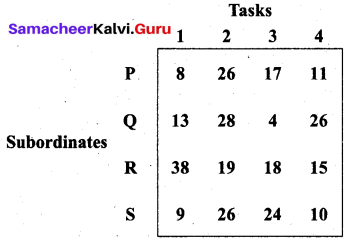How should the tasks be allocated to subordinates so as to minimize the total man-hours?
Solution:
A number of tasks equal the number of subordinates. So the given problem is balanced and we can get an optimal solution.
Step 1: Subtract the minimum hours of each row from other elements of that row.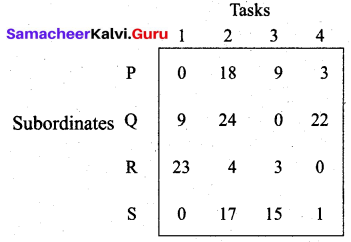Since column 2 has no zero, proceed further.
Step 2: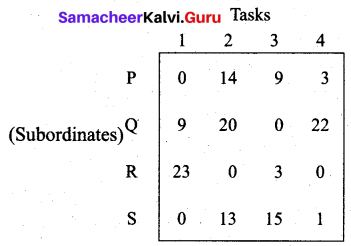We can proceed with the assignment since all the rows and columns have zeros.
Step 3: (Assignment)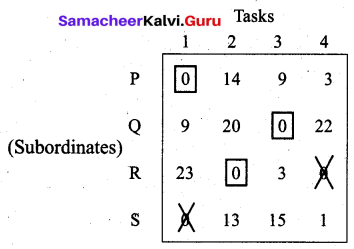Now there is no zero in row S. So we proceed as below.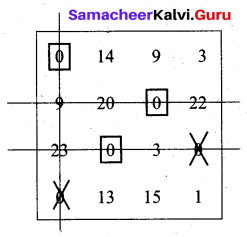We have drawn the minimum number of lines to cover all the zeros in the reduced matrix obtained. The smallest element from all the uncovered elements is 1. We subtract this from all the uncovered elements and add them to the elements which lie at the intersection of two lines. Thus we obtain another reduced problem for fresh assignment.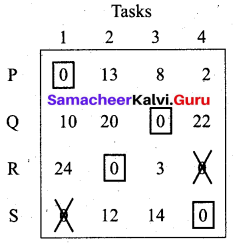Now all the subordinates have been assigned tasks. The optimal assignment schedule and the total cost is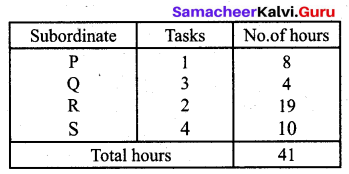The optimal assignment (minimum) hours = 41Question 7.
Find the optimal solution for the assignment problem with the following cost matrix.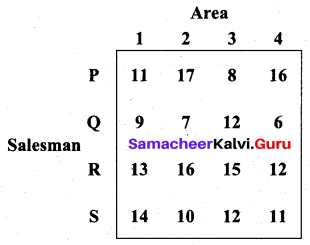Solution:
The number of Areas = Number of salesmen.
So the given problem is balanced and we can find an optimal solution.
Step 1: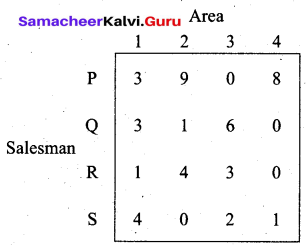Step 2: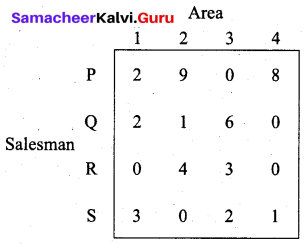Step 3: (Assignment)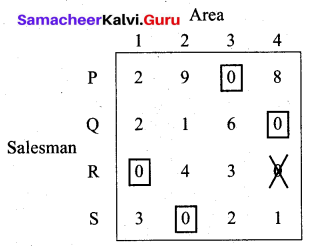Now all the salesmen have been assigned areas.
The optimal assignment schedule and the total cost is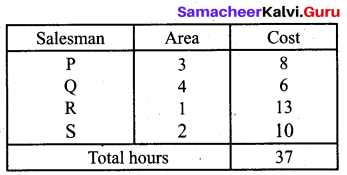Thus the optimal cost is Rs. 37.

Question 8.
Assign four trucks 1, 2, 3, and 4 to vacant spaces A, B, C, D, E, and F so that distance travelled is minimized. The matrix below shows the distance.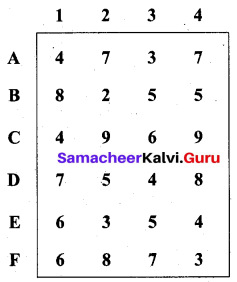Solution:
Here the number of trucks is 4 and vacant spaces are 6. So the given assignment problem is the unbalanced problem. So we introduce two dummy columns with all the entries zero to make it balanced. So the problem is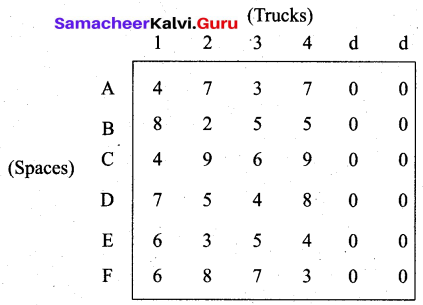Here only 4 vacant spaces can be assigned to four trucks
Step 1: Not necessary since all rows have zeros.
Step 2: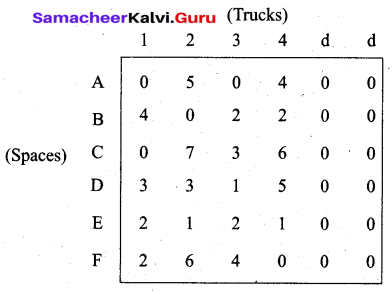Step 3: (Assignment)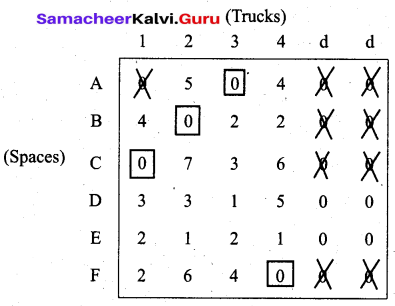The optimal assignment schedule and total distance travelled is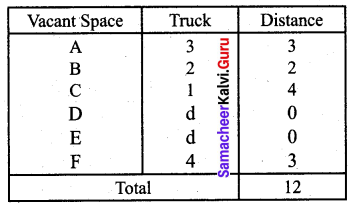Thus the minimum distance travelled is 12 km.